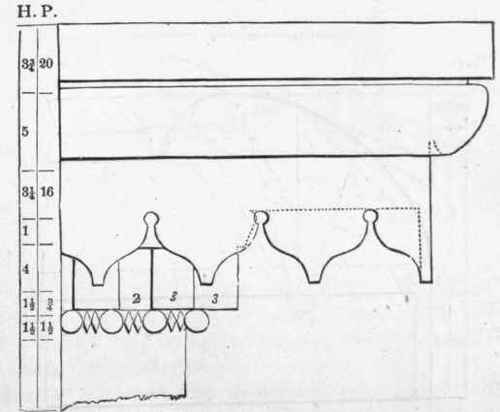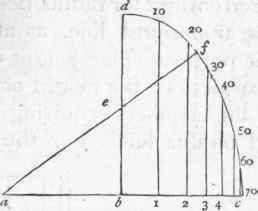Figs. 232 to 240 are designs for eave cornices, and Figs. 241 and 242 are for stucco cornices for the inside finish of rooms. In some of these the projection of the uppermost member from the facia is divided into twenty equal parts, and the various members are proportioned according to those parts, as figured under H and P.Fig. 232.

## 320. - Have Cornices Proportioned To Height Of Building

Draw the line ac (Fig. 243), and make be and ba each equal to 36 inches; from b draw bd at right angles to ac, and equal in length to 3/4 of ac, bisect b d m c, and from a, through e, draw af; upon a, with ac for radius, describe the arc cf, and upon e, with e f for radius, describe the arc fd; divide the curve dfc, into 7 equal parts, as at 10, 20, 30, etc., and from these points of division draw lines to bcFig. 233.Fig. 234.Fig. 235.Fig. 236.Fig. 237.Fig. 238.Fig. 239.Fig. 240.Fig. 241.Fig. 242.

### Proportion Of Cornices

parallel to db; then the distance b 1 is the projection of a cornice for a building 10 feet high; b 2, the projection at 20 feet high; b 3, the projection at 30 feet, etc. If the projection of a cornice for a building 34 feet high is required, divide the arc between 30 and 40 into 10 equal parts, and from the fourth point from 30 draw a line to the base, b c, parallel with b d; then the distance 0/ the point at which that line cuts the base from b will be the projection required. So proceed for a cornice of any height within 70 feet. The above is based on the supposition that 36 inches is the proper projection for a cornice 70 feet high. This, for general purposes, will be found correct; still, the length of the line be may be varied to suit the judgment of those who think differently.Fig. 243.Fig. 244.

Having obtained the projection of a cornice, divide it into 20 equal parts, and apportion the several members according to its destination - as is shown at Figs. 238, 239, and 240.